Next: Visualization of the Wavelet Up: Multiresolution with scaling functions Previous: The Wavelet transform using

### The Reconstruction

If the wavelet is the difference between two resolutions, an evident reconstruction for a wavelet transform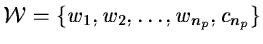is: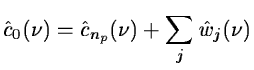(14.60)

But this is a particular case and other wavelet functions can be chosen. The reconstruction can be done step by step, starting from the lowest resolution. At each scale, we have the relations:(14.61)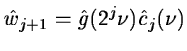(14.62)

we look for cj knowing cj+1, wj+1, h and g. We restore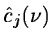with a least mean square estimator: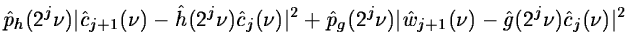(14.63)

is minimum.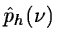and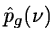are weight functions which permit a general solution to the restoration of. By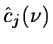derivation we get: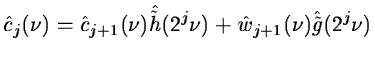(14.64)

where the conjugate filters have the expression: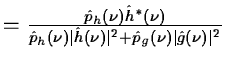(14.65)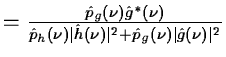(14.66)

It is easy to see that these filters satisfy the exact reconstruction equation 14.19. In fact, equations 14.65 and 14.66 give the general solution to this equation. In this analysis, the Shannon sampling condition is always respected. No aliasing exists, so that the dealiasing condition 14.18 is not necessary.

The denominator is reduced if we choose: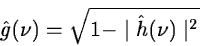This corresponds to the case where the wavelet is the difference between the square of two resolutions:(14.67)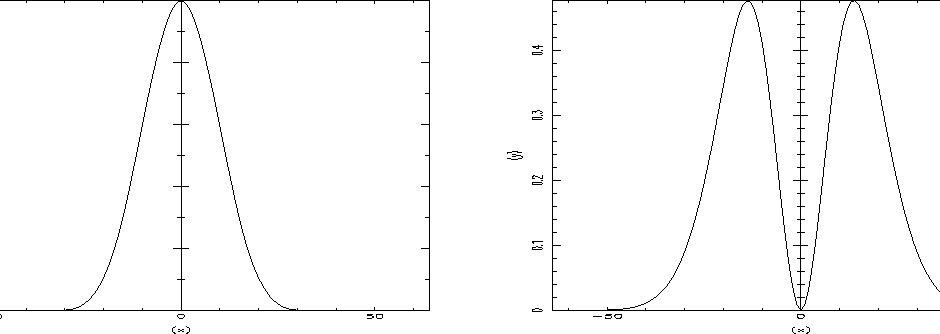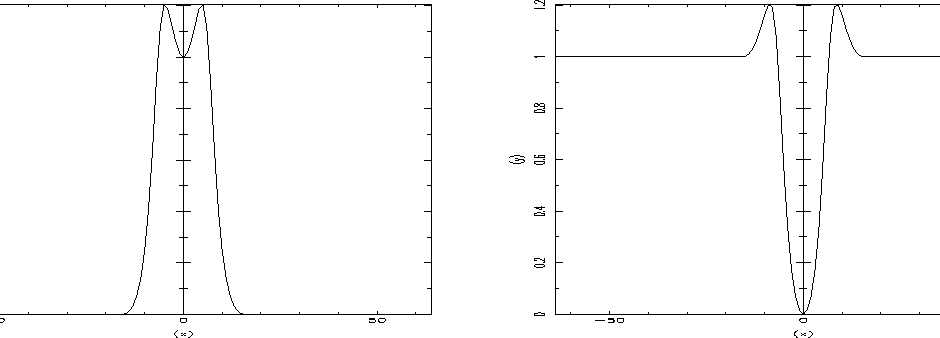We plot in figure 14.10 the chosen scaling function derived from a B-spline of degree 3 in the frequency space and its resulting wavelet function. Their conjugate functions are plotted in figure 14.11.

The reconstruction algorithm is:

1.
We compute the FFT of the image at the low resolution.
2.
We set j to np. We iterate:
3.
We compute the FFT of the wavelet coefficients at the scale j.
4.
We multiply the wavelet coefficients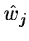by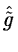.
5.
We multiply the image at the lower resolution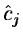by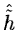.
6.
The inverse Fourier Transform of the addition of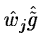and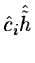gives the image cj-1.
7.
j = j - 1 and we go back to 3.

The use of a scaling function with a cut-off frequency allows a reduction of sampling at each scale, and limits the computing time and the memory size.Next: Visualization of the Wavelet Up: Multiresolution with scaling functions Previous: The Wavelet transform using
Petra Nass
1999-06-15